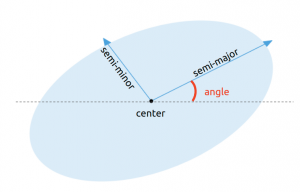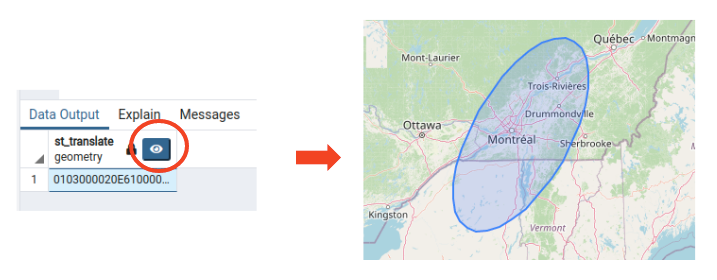# PostGIS: Draw an Ellipse

For the definition of your ellipse, you will need

• The center longitude and latitude
• The length of the semi-major axis and semi-minor axis, in units of the spatial reference system
• The angle of rotation of your ellipse, in radiansTo transform degrees to radians, remember that pi radians = 180°

• your angle in radians = (your angle in degree)*pi/180

For the spatial reference system we are going to use the  EPSG:4326 lat/lon grid. To convert meters to units of this spatial reference system, I use the quick and dirty method from this GIS Stack Exchange post:

• 1 degree of latitude ≃ 111.111 km | that’s your y axis
• 1 degree of longitude ≃ 111,111 * cos(latitude) | that’s your x axis

### The Query

Here is how a SELECT query looks like if you want to get an ellipse

```SELECT
ST_Translate(
ST_Rotate(
ST_Scale(
ST_Buffer(
ST_SetSRID(ST_Point(0,0), 4326),
1.0),
2, 1),
45*pi()/180),
-73.4, 45.4
) ```

And this is the output you would get with PgAdmin### Explanation

1. Take a point at (0,0)  ST_Point(0,0)
2. Set it to WGS84 (SRID 4326)  ST_SetSRID(point, 4326)
3. Add a buffer to make that point a circle, here I’ve added a buffer of 1 degree ST_Buffer(point, 1.0)
4. Scale the circle differently in x and y to make it an ellipse ST_Scale(circle, 2, 1)
5. Rotate your ellipse by the angle, here I am rotating by 45 degrees and turning it into radians ST_Rotate(ellipse, 45*pi()/180)
6. Translate your ellipse to your its center in latitude/longitude ST_Translate(ellipse, -73.4, 45.4)